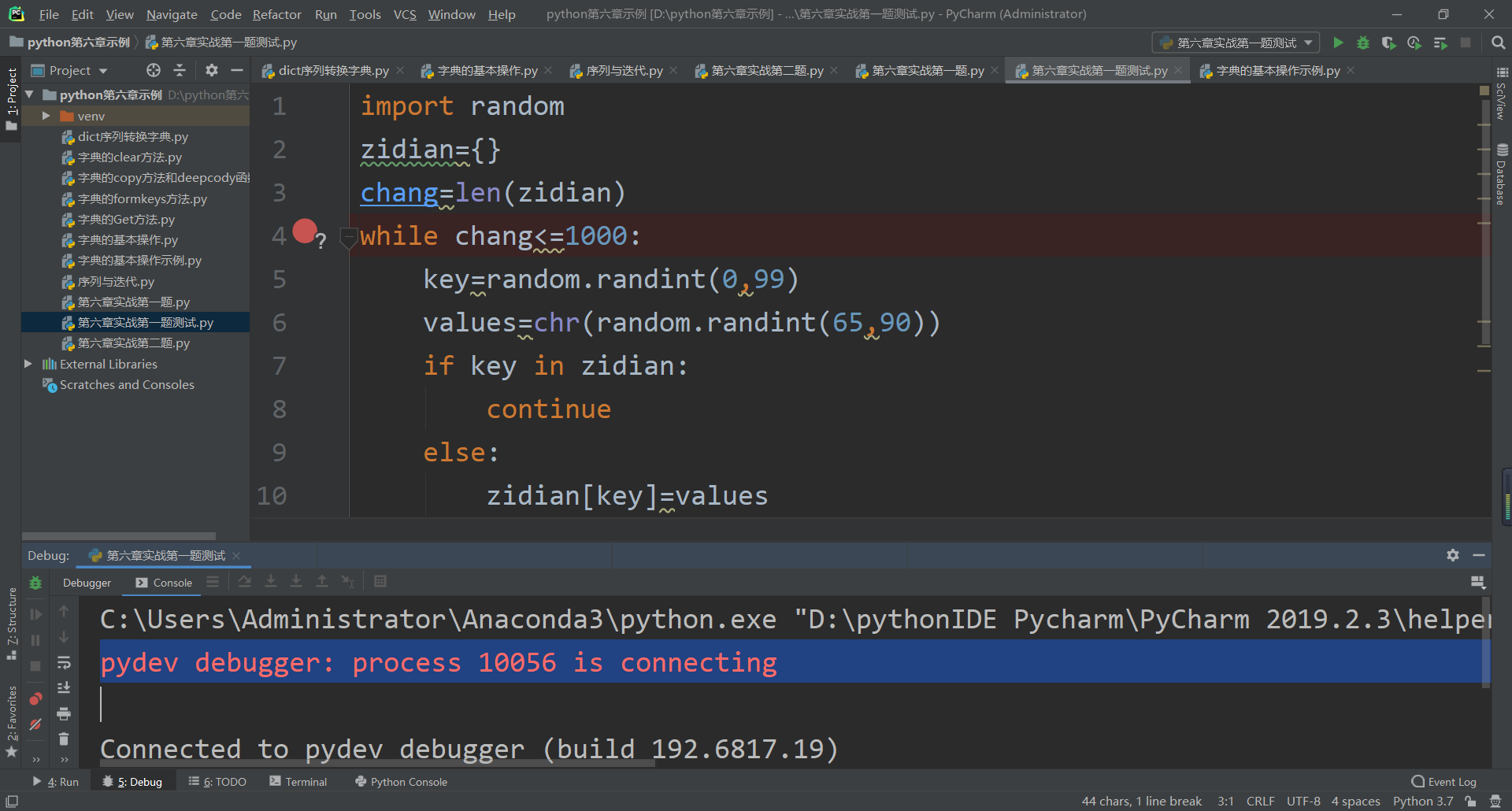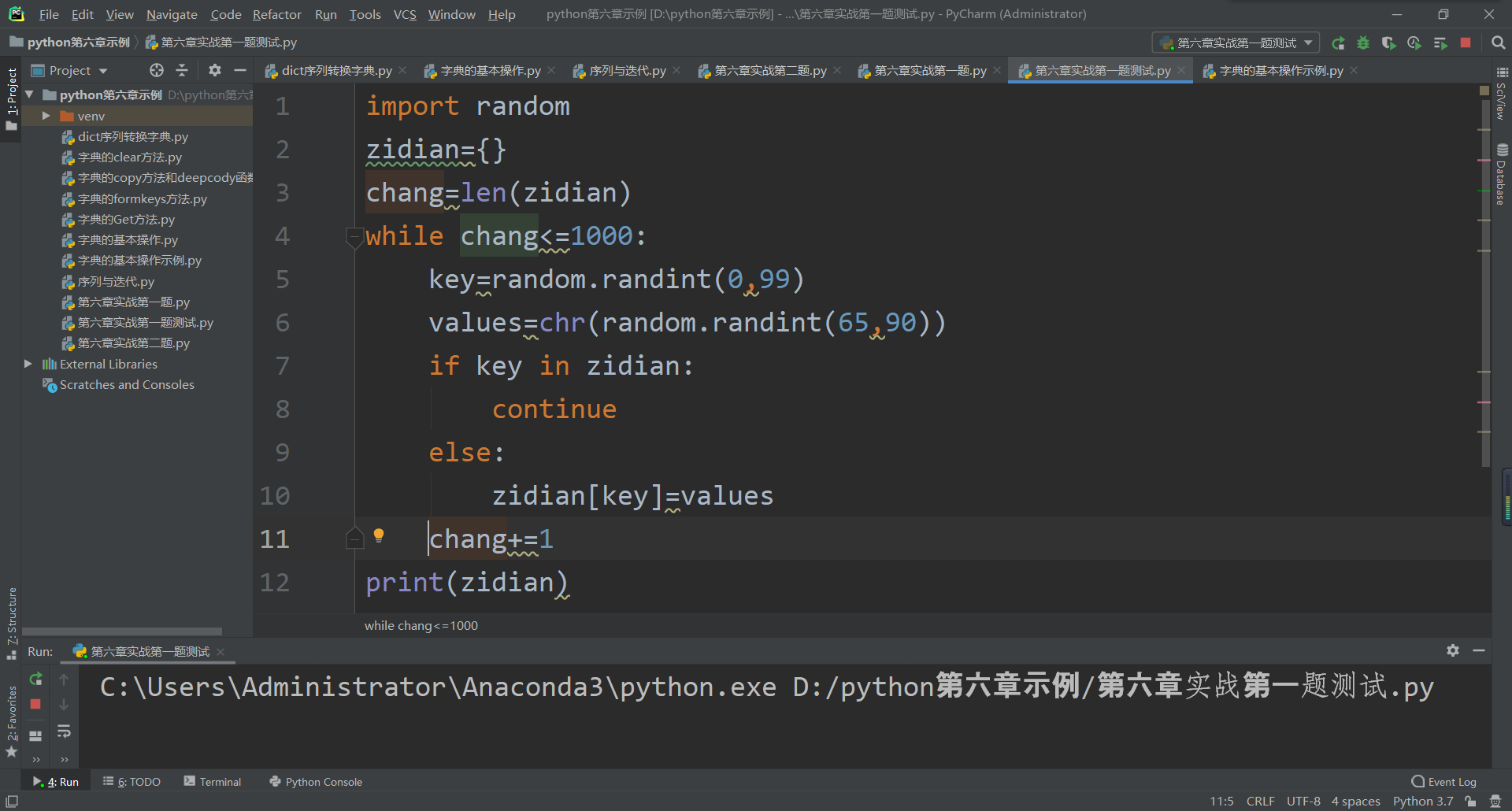python的运行调试问题

import random
zidian={}
chang=len(zidian)
while chang<=1000:
key=random.randint(0,99)
values=chr(random.randint(65,90))
if key in zidian:
continue
else:
zidian[key]=values
chang+=1
print(zidian)

pydev debugger: process 10056 is connecting2个回答

0-99之间的随机int数最高是100个

``````import random
zidian={}
chang=len(zidian)
while chang<=1000:
key=random.randint(0,99)
values=chr(random.randint(65,90))
if key in zidian:
continue
else:
zidian[key]=values
chang+=1
print(zidian)

``````7 个月之前 回复A_B_C_zzy 我加了张图，运行的时候没结果，但是把while改小一点（我试过chang<=10）就出来了，是什么原因，机制的问题吗？
7 个月之前 回复